PWLS-PR: low-dose computed tomography image reconstruction using a patch-based regularization method based on the penalized weighted least squares total variation approachOriginal Article

# PWLS-PR: low-dose computed tomography image reconstruction using a patch-based regularization method based on the penalized weighted least squares total variation approach

Jing Fu1,2#, Fei Feng3#, Huimin Quan2#, Qian Wan1,4, Zixiang Chen1, Xin Liu1, Hairong Zheng1, Dong Liang1, Guanxun Cheng3, Zhanli Hu1^

1Lauterbur Research Center for Biomedical Imaging, Shenzhen Institutes of Advanced Technology, Chinese Academy of Sciences, Shenzhen, China; 2College of Electrical and Information Engineering, Hunan University, Changsha, China; 3Department of Radiology, Peking University Shenzhen Hospital, Shenzhen, China; 4Shenzhen College of Advanced Technology, University of Chinese Academy of Sciences, Shenzhen, China

#These authors contributed equally to this work.

^ORCID: 0000-0003-0618-6240.

Correspondence to: Guanxun Cheng. Department of Radiology, Peking University Shenzhen Hospital, Shenzhen, China. Email: 18903015678@189.cn; Zhanli Hu. Lauterbur Research Center for Biomedical Imaging, Shenzhen Institutes of Advanced Technology, Chinese Academy of Sciences, Shenzhen, China. Email: zl.hu@siat.ac.cn.

Background: Radiation exposure computed tomography (CT) scans and the associated risk of cancer in patients have been major clinical concerns. Existing research can achieve low-dose CT imaging by reducing the X-ray current and the number of projections per rotation of the human body. However, this method may produce excessive noise and fringe artifacts in the traditional filtered back projection (FBP)-reconstructed image.

Methods: To solve this problem, iterative image reconstruction is a promising option to obtain high-quality images from low-dose scans. This paper proposes a patch-based regularization method based on penalized weighted least squares total variation (PWLS-PR) for iterative image reconstruction. This method uses neighborhood patches instead of single pixels to calculate the nonquadratic penalty. The proposed regularization method is more robust than the conventional regularization method in identifying random fluctuations caused by sharp edges and noise. Each iteration of the proposed algorithm can be described in the following three steps: image updating via the total variation based on penalized weighted least squares (PWLS-TV), image smoothing, and pixel-by-pixel image fusion.

Results: Simulation and real-world projection experiments show that the proposed PWLS-PR algorithm achieves a higher image reconstruction performance than similar algorithms. Through the qualitative and quantitative evaluation of simulation experiments, the effectiveness of the method is also verified.

Conclusions: Furthermore, this study shows that the PWLS-PR method reduces the amount of projection data required for repeated CT scans and has the useful potential to reduce the radiation dose in clinical medical applications.

Keywords: Lose-dose computed tomography (lose-dose CT); image reconstruction; patch regularization; penalized weighted least squares (PWLS); total variation (TV)

Submitted Aug 30, 2020. Accepted for publication Feb 01, 2021.

doi: 10.21037/qims-20-963

## Introduction

X-ray computed tomography (CT) has been widely used in the field of diagnosis (1,2). Due to the large dose of radiation received during CT scanning, the potential harm caused by exposure from the radiation has become a public concern (3). Conventional analytical CT algorithms, such as filtered back projection (FBP) is an analytical solution in 2D CT reconstruction and served as the reference. Using these algorithms, the projection must be discretized at a high sampling rate to make the image quality satisfactory, but an excessively high radiation dose will have a negative impact on the health of the patient; in addition, if the sparse view measurement sampling is insufficient, the traditional algorithm FBP method cannot produce acceptable image quality for diagnosis (4-6). Scholars have conducted many studies in recent years, including studies on hardware-based scanning protocols (7-9) and software-based image reconstruction techniques (10-13), to determine how to reduce the radiation dose of CT scans. Low-dose CT imaging can be achieved by reducing the X-ray current (14) and by reducing the number of projections per rotation of the human body (11). However, in the image reconstruction process, the noise created by the insufficient projections will inevitably lead to the degradation of image quality. To date, a variety of low X-ray current or sparse-view CT image reconstruction methods have been proposed. In this paper, based on the existing research, we simulate the use of a newly proposed method based on the data obtained by combining low X-ray current and sparse-view protocols to perform low-dose CT scanning under penalized weighted least squares (PWLS) criterion reconstruction. Then, we focus on reconstructing CT images from the sparse-view projection data for comparison with other existing algorithms.

For reconstruction images using lower dose, many improved methods have been proposed (15-19). The filter-based algorithm is effective and can suppress noise, but due to the lack of noise modeling in the filter, key structural details may be ignored in the reconstruction process (4-6). The statistical iterative reconstruction (SIR) algorithm (20-28), which uses the statistical characteristics of the data, has been proven to perform well in suppressing noise and streak artifacts (29). Statistics-based sinogram restoration and image reconstruction algorithms have shown advantages in reducing noise (30-32). The SIR algorithm uses the advantages of statistical modeling to achieve low-dose CT image reconstruction. The related objective function is generally composed of two terms: a data fidelity term and a penalty term. The data fidelity term is derived from a statistical measurement model; the penalty term is usually designed by considering the properties of the desired image itself. For example, by modeling the characteristics of signal-dependent noise, the PWLS method proposed by Wang et al. shows robustness in the sinogram space and image domain (30).

For sparse-view image reconstruction, Sidky et al. proposed an innovative algorithm based on projections onto convex sets (POCS) (33), which uses the piecewise constant assumption to minimize the total variation (TV) of the image; this method is called the TV-POCS algorithm (34,35). An adaptive-steepest-descent-based POCS (ASD-POCS) method based on the updated TV-POCS algorithm, which minimizes the TV in sparse-view CT image reconstruction and improves the denoise performance and suppresses cone-beam artifacts, was proposed (36). The ASD-POCS algorithm performed well in suppressing cone-beam artifacts generated by sparse projection data (36). However, the characteristics the peripheral part of the image in the TV minimization algorithm are isotropic, so related algorithms often produce an over-smoothing effect. Therefore, a weighted TV minimization approach, as an extension of the conventional TV minimization approach, is proposed to solve the abovementioned problems in sparse-view CT image reconstruction (37,38). For example, Zhang et al. proposed using restored sinogram data for image reconstruction based on the PWLS-based TV (PWLS-TV) minimization method, which outperforms the PWLS method (39).

The local patch-based processing method has attracted more attentions, and it has been proven to be an effective method for preserving the patch characteristics. Patch-based methods can obtain more image features than pixel-based methods, and they have been widely used in image denoising, restoration and reconstruction in recent years (40-42). The traditional nonquadratic penalty algorithm uses the difference of the intensity between adjacent pixels to calculate the roughness on the image, which increases the robustness of the algorithm. If the image has serious noise, edge detection will be unreliable. The difference in the intensities between adjacent pixels is can be used to evaluate the results of edge detection in images affected by noise. To overcome this problem, Wang et al. proposed a patch-based regularization method that uses neighborhood patches instead of individual pixels to measure image roughness (40). Patch-based regularization comparing the similarity between each patch outperform the pixel-based regularization (40). Kervrann et al. proposed a novel adaptive patch-based approach for image denoising and representation (43). Wang and Qi developed a patch-based regularization method for iterative image reconstruction, which uses neighborhood patches instead of individual pixels to calculate the nonquadratic penalty (40). Patch-based reconstruction methods are also more robust to hyperparameter selection than traditional pixel-based reconstruction methods (44). Lu et al. proposed a dictionary-based method to process an input image patch by patch, unlike conventional image processing techniques that handle an image pixel by pixel (45). The reconstructed images obtained by using this approach are significantly better than those by using approaches based on pixel-by-pixel processing (46,47).

Inspired by the ideas of the above methods, we are motivated to study whether the image quality can be further improved by combining the patch-based regularization method with the PWLS-TV algorithm. Thus, we propose the PWLS-PR method. Each iteration of the proposed algorithm can be described in the following three steps: image updating via the PWLS-TV, image smoothing, and pixel-by-pixel image fusion. In simulation experiments and physical experiments, qualitative and quantitative evaluations were measured on CT images reconstructed from sparse-view acquisitions. For undersampling and noisy image reconstruction, the experimental results show that this method can preserve more details than other methods while reducing noise and suppressing artifacts.

This paper is organized as follows. We introduce the related theory and describe the proposed algorithm in detail in the “Methods” section. The description of the simulation experiments and the image quality metrics used are presented in the “Computer simulation” section. The physical experiment is presented in the “Physical experiment” section. A discussion of the results is presented in the “Discussion” section, and the conclusions are presented in the “Conclusions” section.

## Methods

### CT imaging model

Under the assumption of a single energy beam, an X-ray CT measurement can be approximated by the following discrete linear system (39):

$y=Hμ$

where y=(y1, y2, …yM)T represents the sinogram data obtained following prediction system calibration and logarithmic transformation and the superscript T represents the matrix transpose. H represents the M×N system or projection matrix that can be calculated in advance by fast ray tracing technology. µ = (µ1, µ2, , µN)T represents the attenuation coefficient estimate of the vector.

When there is a measurement deviation or additional noise, the X-ray CT measurement can be expressed by the following formula:

$y=Hμ+e$

where e represents measurement deviation and additional noise.

The goal of CT image reconstruction is to use the system or projection matrix H to estimate the attenuation coefficient µ from the sinogram data y.

### Existing methods of CT image reconstruction

#### PWLS method

Based on the existing research, the PWLS criterion for CT image reconstruction can be rewritten as follows (32):

$p * = argmin u≥0 { ( y−p ) ′ ∑ -1 ( y−p )+βR( p ) }$

where y=(y1, y2, ..., yM)T represents the sinogram data and p represents the ideal projection vector to be estimated. Σ is a diagonal matrix whose ith element is, which is the variance of the sinogram data y. β is a hyperparameter used to balance the fidelity term and the a priori penalty term. R(p) is the image roughness penalty.

Conventionally, the image roughness is measured based on the intensity difference between neighboring pixels (31). In the PWLS method, R(p) uses the following form of a quadratic penalty:

$R( p )= 1 2 ∑ j ∑ k∈ M j ω j,k ( p j − p k ) 2$

where Mj represents the set of four nearest neighbors of the jth voxel in the sinogram. ωj,k is the weighting coefficient related to the distance between pixels j and k in Mj. The weighting coefficient ωj,k is considered to be inversely proportional to the Euclidean distance between two pixels.

Based on previous research, the mathematical expression proposed by Huang et al. (48) can be used to calculate the variance as follows:

$σ i 2 = 1 I 0 exp( p i ¯ )( 1+ 1 I 0 exp( p i ¯ )( σ e 2 −1.25 ) )$

where I0 represents the X-ray intensity, represents the mean of the sinogram data in bin i and $σe2$ is the variance of the background electronic noise.

#### PWLS-TV method

When the dimensionality of the system matrix in the CT system is very large, and when reconstruction is performed in the sinogram data of the measurement noise, the dimensionality of the system matrix will be seriously degraded, and it will be very difficult to directly calculate the attenuation coefficient estimate µ in equation (2). Therefore, in this paper, we use the PWLS-based criterion, which can be expressed as follows (48):

$μ*= argmin μ≥0 { ( p−Hμ ) ′ ∑ −1 ( p−Hμ )+βR( μ ) }$

where H represents the system or projection matrix, p represents the restored sinogram data from equation (3), and µ represents the attenuation coefficient to be estimated. Σ represents a diagonal matrix whose ith element is, which is defined in formula (5). β represents a hyperparameter used to balance the fidelity term (the first term on the right side of Eq. ) and the penalty term (the second term on the right side of Eq. ). For the PWLS-TV and PWLS-PR algorithms proposed later, an identical value of β can be used.

In this paper, a TV-based penalty term is used, which can be written as (49):

$R( μ )= ∑ v,w ( μ v,w − μ v−1,w ) 2 + ( μ v,w − μ v,w−1 ) 2 +δ$

where v and w represent the position indicators of the attenuation coefficient of the desired image. δ is a small constant used to ensure that this term is differentiable anywhere relative to the voxel value.

### Proposed CT image reconstruction method

When an image is noisy, pixel intensity differences are not a reliable means of distinguishing real edges from noise fluctuations. To overcome this problem, inspired by the penalized likelihood method of positron emission tomography (PET) image reconstruction using patch-based regularization (42), we propose the PWLS-PR method, which uses neighborhood patches instead of individual pixels to measure the image roughness. The proposed method can achieve good results in artifact suppression and structure preservation, and because it compares the similarity between patches, the proposed method is more reliable than the comparison methods PWLS and PWLS-TV.

#### Definition

Traditionally, the image roughness is measured based on the intensity difference between adjacent pixels (40):

$U( μ )= 1 4 ∑ j=1 n j ∑ k∈ N j ω jk ψ( μ j − μ k )$

where U(µ) represents the image roughness penalty and ψ(x) represents the penalty function. wj,k represents the weighting factor related to the distance between pixels j and k in neighborhood Nj.

We add this image roughness penalty term to the PWLS-TV method to obtain our proposed PWLS-PR method. The associated mathematical formula for the PWLS-PR method can be expressed as follows:

$μ*= argmin μ≥0 { ( p−Hμ ) ′ ∑ −1 ( p−Hμ )+βR( μ ) }−αU( μ )$

where α is a regularization parameter that controls the balance between the data fidelity and spatial smoothness. Too much α value reduces noise and makes the reconstructed image smoother but also reduces the resolution, so the choice of α value is very important for the PWLS-PR algorithm.

Inspired by the penalized likelihood method of PET image reconstruction using patch-based regularization (41), we propose using a patch associated with each pixel to calculate the image roughness between neighboring pixels j and k.

In this paper, the patch of a pixel is a square area with the pixel as the center, and the sizes of all the patches in an image are the same. We can understand the meaning of patch as shown in Figure 1. The proposed patch-based roughness function is defined as follows (40):

$U( μ )= 1 4 ∑ j=1 n j ∑ k∈ Ν j ψ( ‖ f j ( μ )− f k ( μ ) ‖ h )$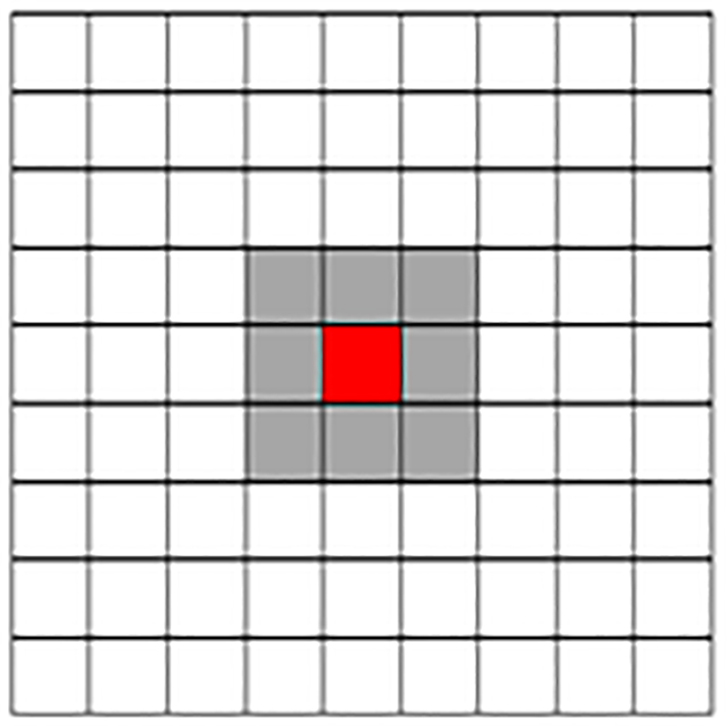Figure 1 An example of a sampling window designed for a single pixel (the central red pixel). The white window corresponds to a patch of size 9×9, and the gray window corresponds to a patch of size 3×3.

where fj(µ) represents the feature vector of the intensity values of all pixels in the patch centered on pixel j. The patch-based distance between pixels j and k is calculated by the following formula:

$‖ f j ( μ )− f k ( μ ) ‖ h = ∑ l=1 m l r l ( μ jl − μ kl ) 2$

where jl represents the lth pixel in the patch associated with pixel j. kl represents the lth pixel in the patch associated with pixel k. ml represents the total number of pixels in a patch. rl represents a positive weighting coefficient equal to the normalized inverse spatial distance between pixel jl and pixel kl.

$∑ l=1 m l r l =1$

From the existing research, we need to obtain a convex penalty function to guarantee the convergence of the proposed algorithm. Then, the penalty function must meet the following three conditions (50):

• The penalty function must be differentiable and symmetric everywhere.
• The first-order derivative must be nondecreasing:

$\stackrel{˙}{\psi }\left(x\right)\triangleq \frac{d\psi \left(x\right)}{dx}$

• When x≥0 and 0 <ωψ(0) < +∞, the curve must be nonincreasing:

${\omega }^{\psi }\left(x\right)\triangleq \frac{d\stackrel{˙}{\psi }\left(x\right)}{dx}$

As discussed in a previous article, examples of possible penalty functions include the following: quadratic functions, the Lange function, the Huber function, and hyperbolic functions (40). When the penalty function is a quadratic function, patch-based regularization is equivalent to pixel-based quadratic regularization. However, the main disadvantage of quadratic regularization is that the discontinuity of the image cannot be taken into account, which may result in excessively smooth edges or fine structures in the reconstructed image.

Based on existing research experience, in this paper, the Lange function is used as the penalty function (40,49):

$ψ( x )=δ( | x | δ −log( 1+ | x | δ ) )$

When |x|<<δ, the function approximates the quadratic function, and when |x|>> δ, the function approaches the absolute function.

#### The proposed patch-based regularization method based on the PWLS-TV (PWLS-PR) algorithm for CT image reconstruction

Due to the nonlinear form of the filter relative to the image intensity, it is difficult for general optimization algorithms to effectively minimize the objective function in equation (9). To solve this problem, in this paper, similar to our previous work (31,48,51), an alternating minimization scheme is used in equation (9), where the weights can be automatically updated based on the similarity between patch windows. The current estimate during each iteration is µn, where n is the iteration index.

The proposed algorithm will guarantee a monotonic convergence to the global solution when the penalty function satisfies the three conditions given in the “Proposed CT image reconstruction method” section. Based on existing research experience, the Lange function is used as the penalty function in this paper, which satisfies the three conditions (40). Therefore, the proposed algorithm is convergent. The whole algorithm is summarized in Algorithm 1 (Table 1).

### Computer simulation

In this study, experimental data were utilized to validate the proposed PWLS-PR algorithm and to compare it with the PWLS algorithm, the PWLS-TV algorithm and the classical FBP algorithm.

The preliminary image reconstructed using the FBP method with a ramp filter was used as the initial estimate for all the algorithms. The total number of iterations n was set to 100.

#### Experimental data acquisition

To verify the performance of the proposed method, we use the digital extended cardiac-torso (XCAT) phantom (52) shown in Figure 2 as analog data for experiments and perform ultra-low-dose image simulation on these analog data under different X-ray current levels and different numbers of views.

The phantom shown in Figure 2 is composed of 512×512 square pixels with intensity values between 0 and 130. The size of each pixel is 0.85 mm × 0.85 mm. We use a representative geometrical figure in CT for scanning and a monoenergetic fan-beam CT scanner setup for a circular orbit. Each rotation included 360 projection views evenly spaced on a circular orbit, and each view contained 672 data elements each from 1 of the 672 detector bins. The distance from the X-ray source to the detector was 1,040 mm, and the distance from the center of rotation to the curved detector was 570 mm.

Each projection datum passing through the tomographic image along the X-ray can be calculated based on the known density and the area of the intersection of the ray and the geometric shape of the object in the tomographic image.

#### Simulation setup

Simulating low-dose noisy projection data is similar to the research in (53). First, the y value of the noise-free line integral is calculated according to Equation  as a direct projection operation, and then the noise measurement value bi in each bin i is calculated according to the following formula for the statistical model of the prelogarithmic projection data:

$b i =Poisson( I 0 exp( − y i ) )+Normal( 0, σ e 2 )$

where I0 represents the incident X-ray intensity and represents the background electronic noise variance, which was set to 10 in this paper.

In this paper, the simulation experiment performed ultra-low-dose CT images under different X-ray current levels and different numbers of views. When simulating different X-ray current experiments, the X-ray exposure level I0 was set to three different levels: 1×105, 1×106 and 5×106. The noisy measurement yi value was calculated through the logarithmic transformation of bi. When the different sparse-view projection data simulation experiments were carried out, the original 360 views were under-sampled with 60, 90, 120, and 180 views.

#### XCAT phantom studies

For image reconstruction under different sparse-view projection data levels, we set the X-ray intensity to a fixed value and reconstructed the images from ultra-low-dose sinogram data obtained with different algorithms at different sparse-view levels. Then, we compare the low-dose reconstruction effect maps of the PWLS, PWLS-TV, and PWLS-PR algorithms for the different numbers of sparse views. As shown in Figure 3, under a fixed I0 =5×106, the number of sparse views from top to bottom is 60, 90, 120, and 180.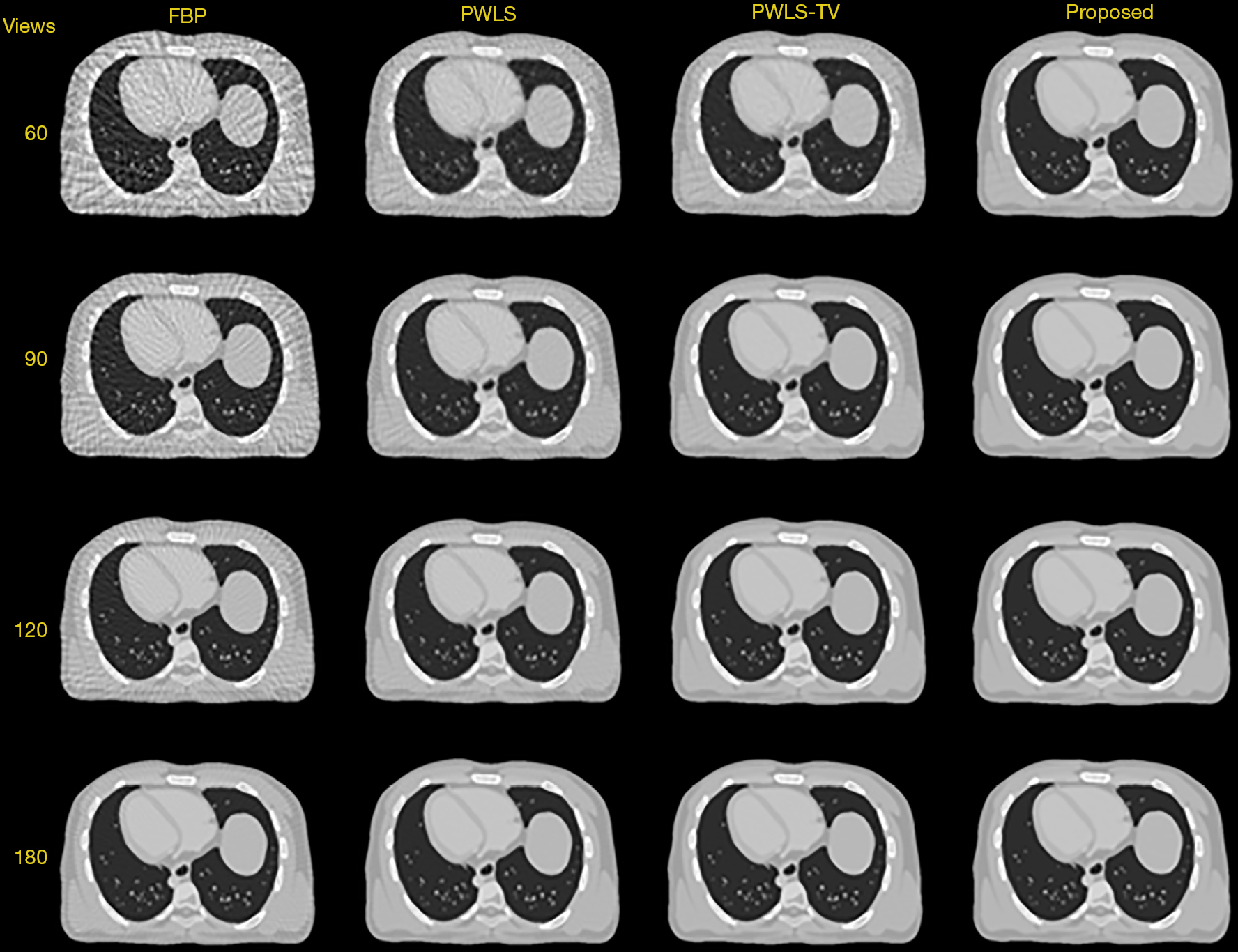Figure 3 XCAT phantom reconstructions using the different methods for a fixed X-ray current level of I0 =5×106 and 60, 90, 120 and 180 views. FBP, filtered back projection; PWLS, penalized weighted least squares; PWLS-TV, total variation based on penalized weighted least squares.

To analyze the reconstruction image in Figure 3, the images reconstructed from the projection data using the FBP approach with a ramp filter were used as the prior images. Obvious differences can be observed between the initial images obtained using the FBP method (shown in the first column in Figure 3) and the desired image (shown in Figure 2). The measurements of the sparse-view projection data resulted in serious fringe artifacts using FBP-based reconstruction. The first column in Figure 3 shows the images reconstructed using FBP with a ramp filter from the 60-, 90-, 120- and 180-view projection data, which were used as the initial estimates for all the iterative algorithms for reconstruction from the same numbers of views. The second and third columns in Figure 3 show the images reconstructed using the PWLS and PWLS-TV approaches, respectively. The fourth column shows the images reconstructed using the proposed PWLS-PR approach. Through the comparison of reconstruction images of different algorithms, it can be seen that the PWLS-PR method has better image recovery effects than the other methods in terms of noise removal and artifact suppression.

For the image reconstruction under different X-ray current levels, we set a fixed number of sparse views and images reconstructed from the ultra-low-dose sinogram data obtained with different algorithms at different X-ray current levels. As shown in Figure 4, under a fixed sparse view =90, the different X-ray current levels from top to bottom are I0 =1×105, I0 =1×106 and I0 =5×106, respectively. The proposed PWLS-PR method also achieves better image recovery than the other methods at each X-ray current level.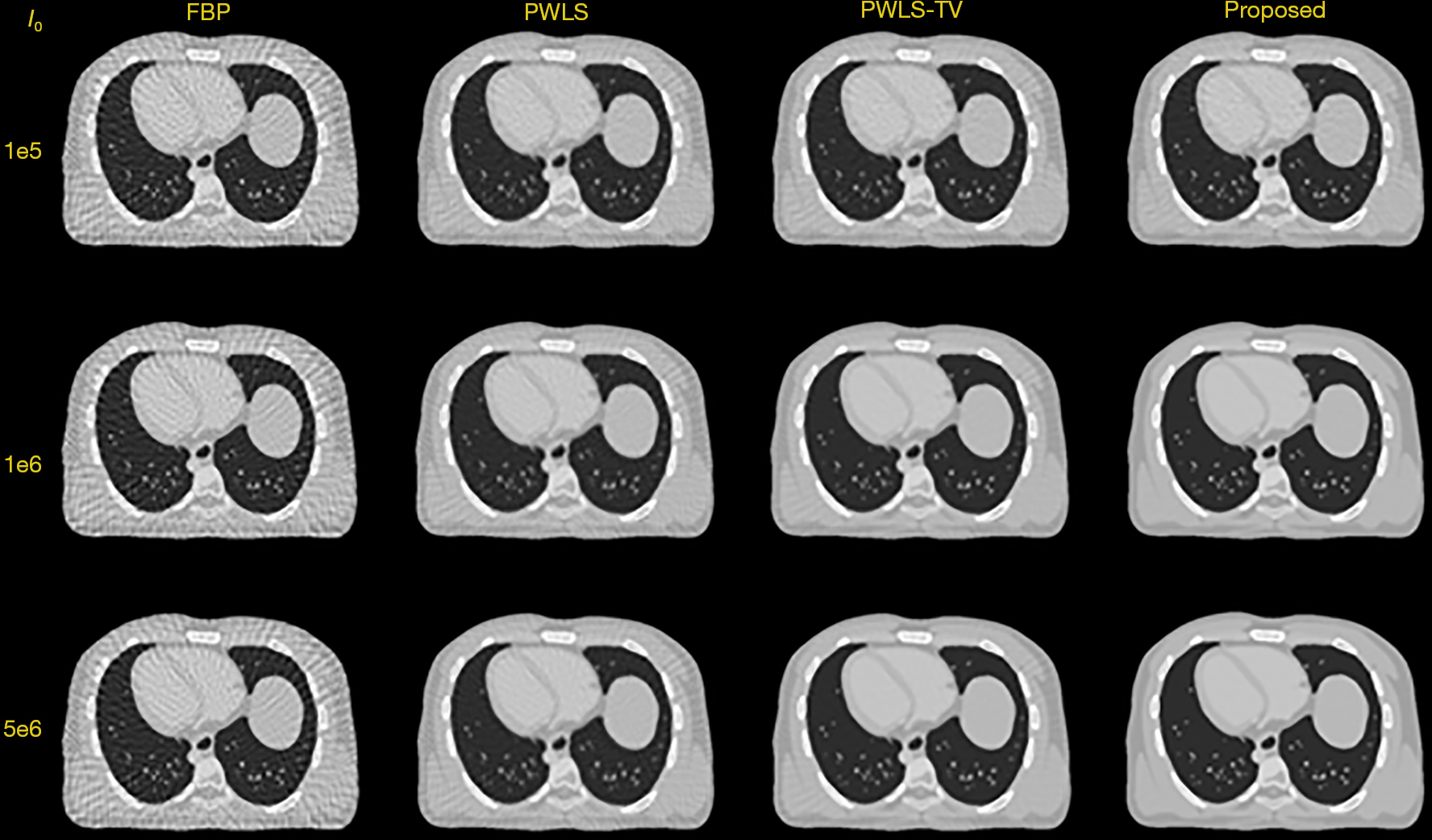Figure 4 XCAT phantom reconstructions using the different methods for 90 views and different X-ray current levels of I0 =1×105, I0 =1×106 and I0 =5×106. FBP, filtered back projection; PWLS, penalized weighted least squares; PWLS-TV, total variation based on penalized weighted least squares.

In Figures 3,4, the results obtained by the FBP, PWLS, PWLS-TV and PWLS-PR methods are shown from left to right. The reconstruction parameter value β of the PWLS, PWLS-TV and PWLS-PR methods is 0.01. The image reconstructed by FBP has serious streak artifacts. It can be seen that the proposed PWLS-PR method has better image recovery effects than other methods in terms of noise removal and artifact suppression.

To further prove the excellent performance of the PWLS, PWLS-TV and PWLS-PR methods, we compared the performance of these three algorithms in reconstructing detailed regions of interest (ROIs). We choose the sparse-view level of 90 views over 2π and the X-ray exposure level of I0 =5×106; the three selected ROIs are shown in Figure 5A. We choose the image reconstruction from the 360 views over 2π at an X-ray exposure level of I0 =1×105, the three selected ROIs are shown in Figure 5B. Zoomed-in views of these three ROIs in Figure 5A are displayed in Figure 6A. Each row in Figure 6A shows the results reconstructed using the different approaches from the 90-view projection data. The image reconstructed from the projection data using the FBP approach with a ramp filter was again used as the initial image. The first row in Figure 6A shows the three ROIs obtained using the PWLS approach, which still exhibit serious streak artifacts. The second and third rows in Figure 6A show the three selected ROIs with detailed structures reconstructed using the PWLS-TV and PWLS-PR approaches, respectively. Additionally, the corresponding zoomed-in views of the three ROIs in Figure 5B are displayed in Figure 6B. All the experimental results prove that the PWLS-PR method achieves remarkable gains over the PWLS and PWLS-TV methods in terms of preserving the structural information of the ROIs.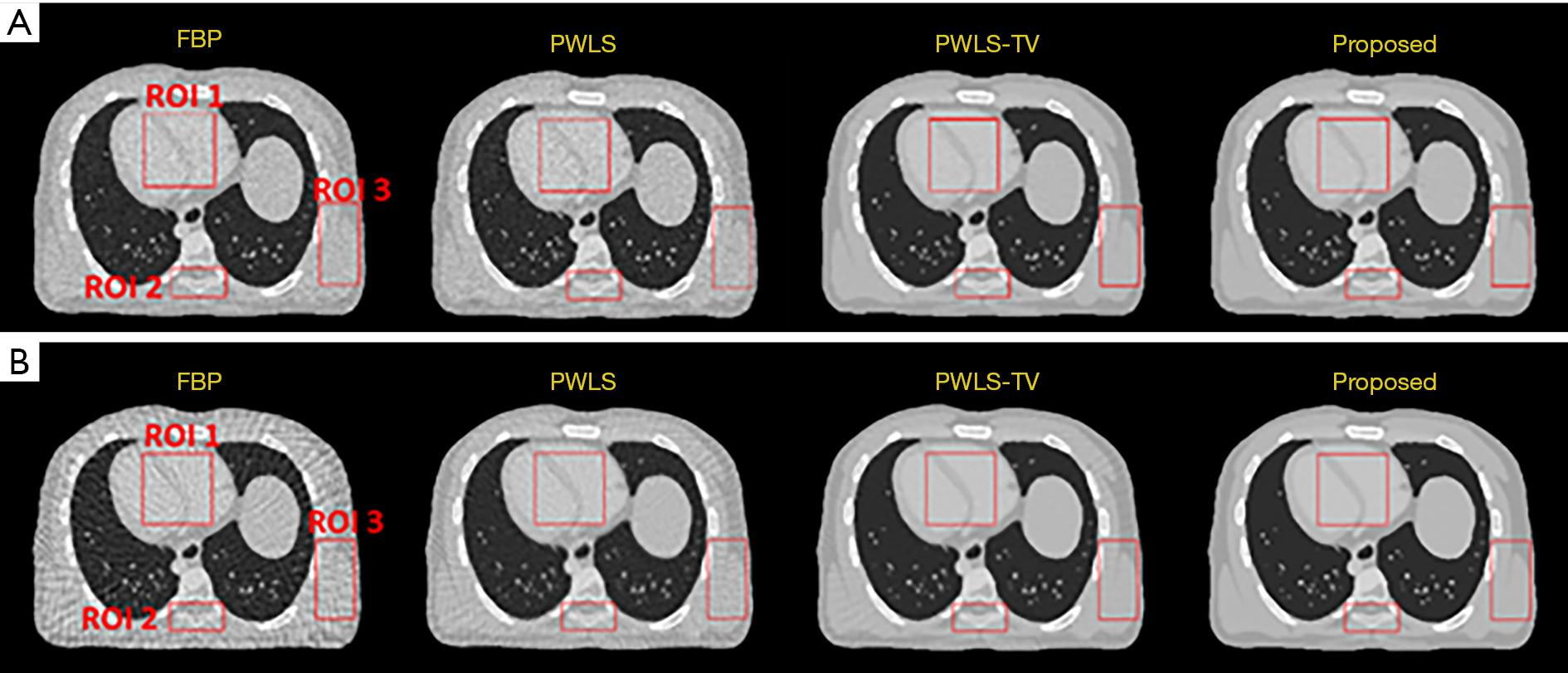Figure 5 Three selected ROIs in the same locations indicated on the results of the PWLS, PWLS-TV and PWLS-PR approaches. (A) Image reconstruction from 90-view projection data over 2π at an X-ray exposure level of I0 =5×106. (B) Image reconstruction from the 360 views over 2π at an X-ray exposure level of I0 =1×105 (in each case, the image reconstructed via FBP from the projection data was used as the initial image). FBP, filtered back projection; PWLS, penalized weighted least squares; PWLS-TV, total variation based on penalized weighted least squares; ROIs, regions of interest; PWLS-PR, patch-based regularization method based on penalized weighted least squares total variation.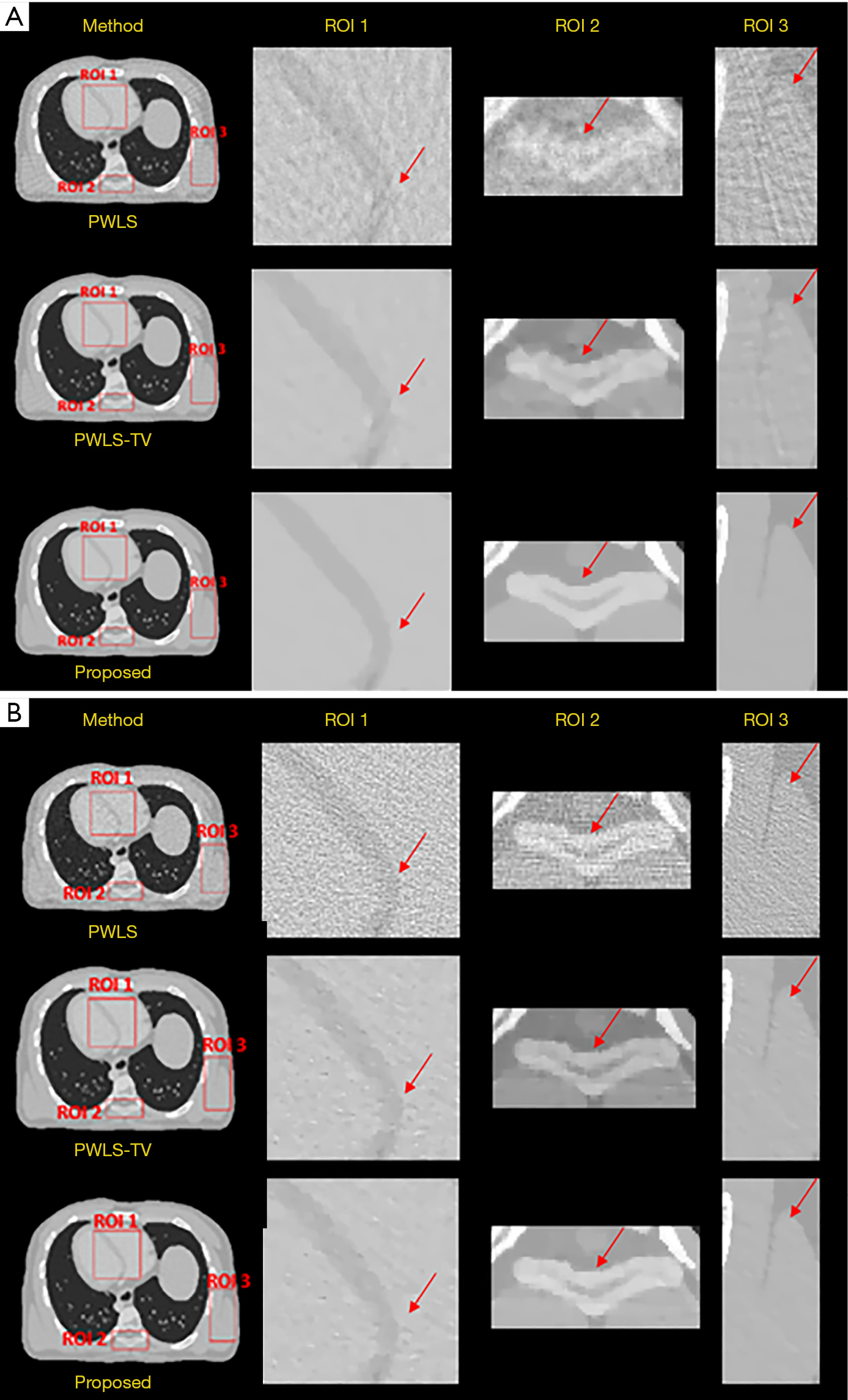Figure 6 Zoomed-in views of the three selected ROIs for the comparison of the PWLS, PWLS-TV and PWLS-PR approaches. (A) Image reconstruction from the 90-view projection data over 2π at an X-ray exposure level of I0 =5×106. (B) Image reconstruction from the 360 views over 2π at an X-ray exposure level of I0 =1×105. FBP, filtered back projection; PWLS, penalized weighted least squares; PWLS-TV, total variation based on penalized weighted least squares; ROIs, regions of interest; PWLS-PR, patch-based regularization method based on penalized weighted least squares total variation.

#### Performance evaluation

To further demonstrate the benefits of our proposed scheme, we quantitatively compare the performance of the PWLS, PWLS-TV and PWLS-PR algorithms on the reconstruction of the whole image and of the ROIs with detailed structures. The peak signal-to-noise ratio (PSNR), the root mean square error (RMSE) and the structural similarity (SSIM) have all been widely used in evaluating the quality of reconstructed CT images (54).

To quantitatively evaluate the PWLS-PR method under different numbers of views and different X-ray current levels, the PSNR, RMSE and SSIM were calculated as image quality indicators for the whole image and for the ROIs indicated by the boxes in Figure 5.

For a fixed X-ray current level of I0 =5×106 and different sparse-view levels of 60, 90, 120 and 180 views, the whole-image results are given in Table 2. In addition to the X-ray current level shown in Figure 3, the X-ray exposure level I0 was set to two other values, i.e., 1×105 and 1×106, and a fixed sparse-view level of 90 views was used. The corresponding whole-image results are listed in Table 3. It can be seen from these tables that the PWLS-PR method outperforms the PWLS and PWLS-TV methods in most cases, especially when the radiation dose is lower.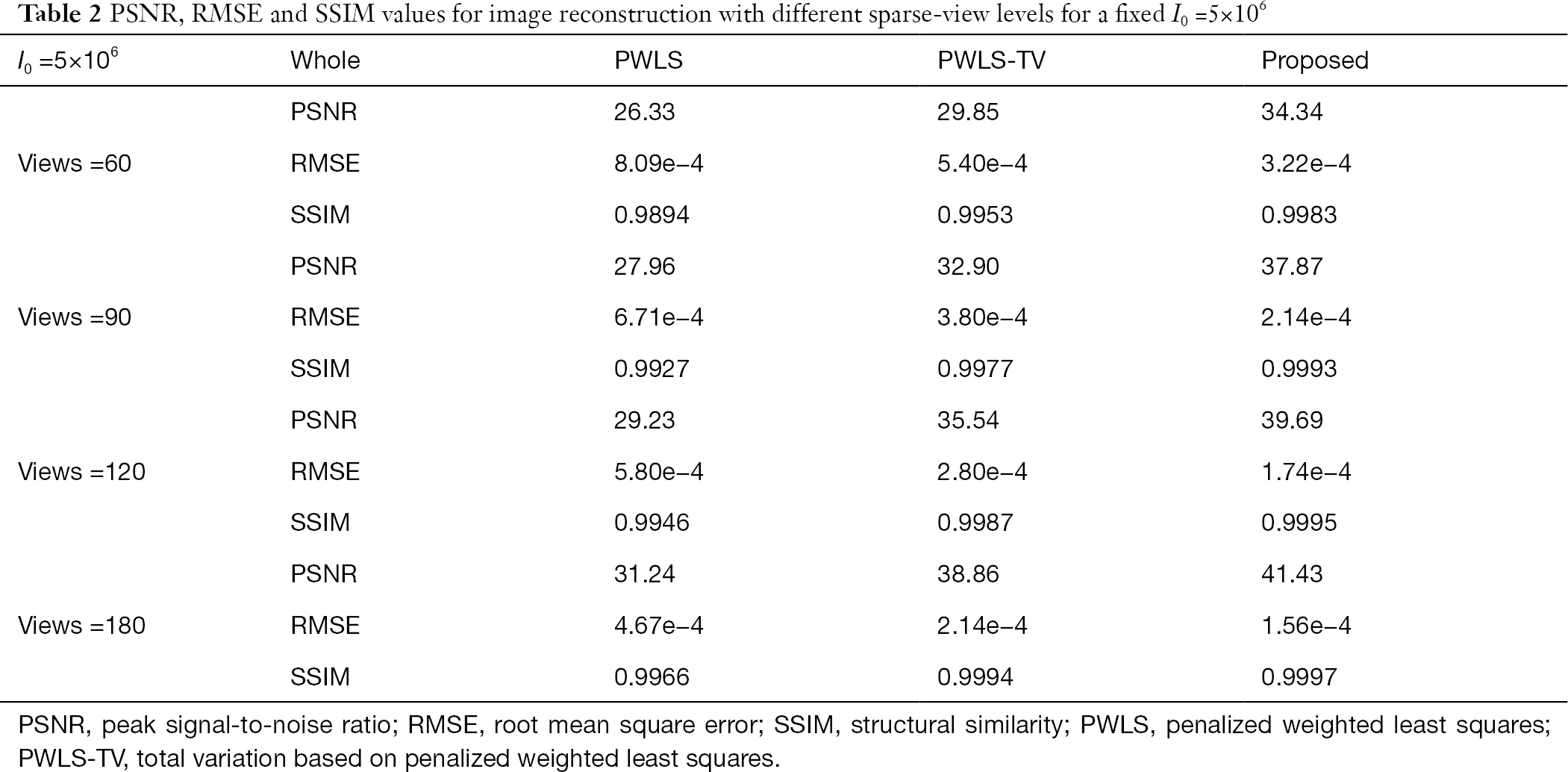Table 2 PSNR, RMSE and SSIM values for image reconstruction with different sparse-view levels for a fixed I0 =5×106
Full table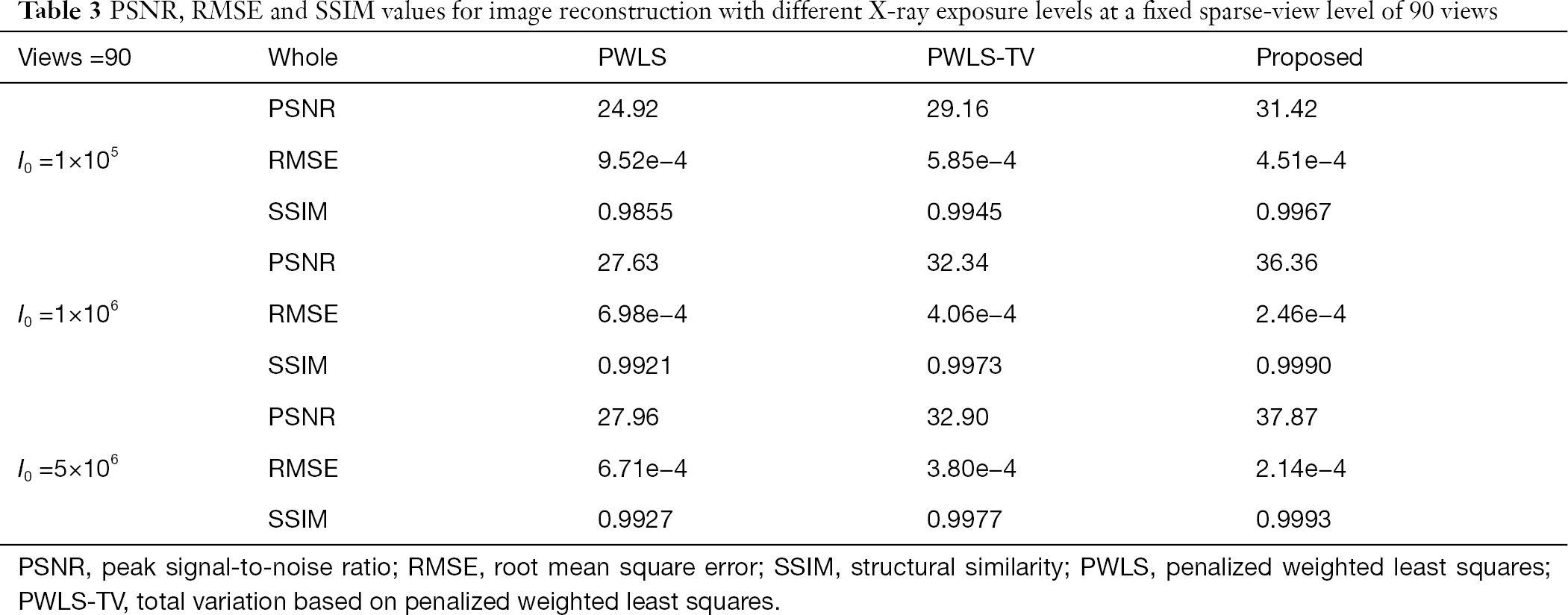Table 3 PSNR, RMSE and SSIM values for image reconstruction with different X-ray exposure levels at a fixed sparse-view level of 90 views
Full table

To more clearly compare the PSNR, RMSE and SSIM values for low-dose CT image reconstruction, these metrics were also computed for the three ROIs marked with red boxes in Figure 5A. The results are shown in Figure 7. We can conclude that PWLS-PR outperforms the PWLS-TV method by more than 15% and outperforms the PWLS method by more than 30% in terms of the PSNR, while the RMSE of PWLS-PR is the lowest among the three methods. A lower RMSE value and a higher PSNR value indicate better-quality reconstructed images. In addition, a higher SSIM value indicates that the reconstructed images are more similar to the reference images in terms of the structure and perceptual features. Through comparisons, it can be seen that the images processed by the PWLS-PR method are also the closest to the reference images. We describe the intensity-pixel position map of these algorithms in Figure 8. It can be found that the red line of the PWLS-PR algorithm is the closest to the black line of the reference image. This shows that the reconstructed image obtained by the PWLS-PR algorithm is the closest to the original image. In addition, we compare the performance of these algorithms through residual graphs. As shown in Figure 9, it can be known that the noise and artifacts in Figure 9D are the least. This means that compared with other algorithms to reconstruct images, the reconstructed image obtained by the PWLS-PR algorithm has the least noise and artifacts, and the algorithm has the highest robustness.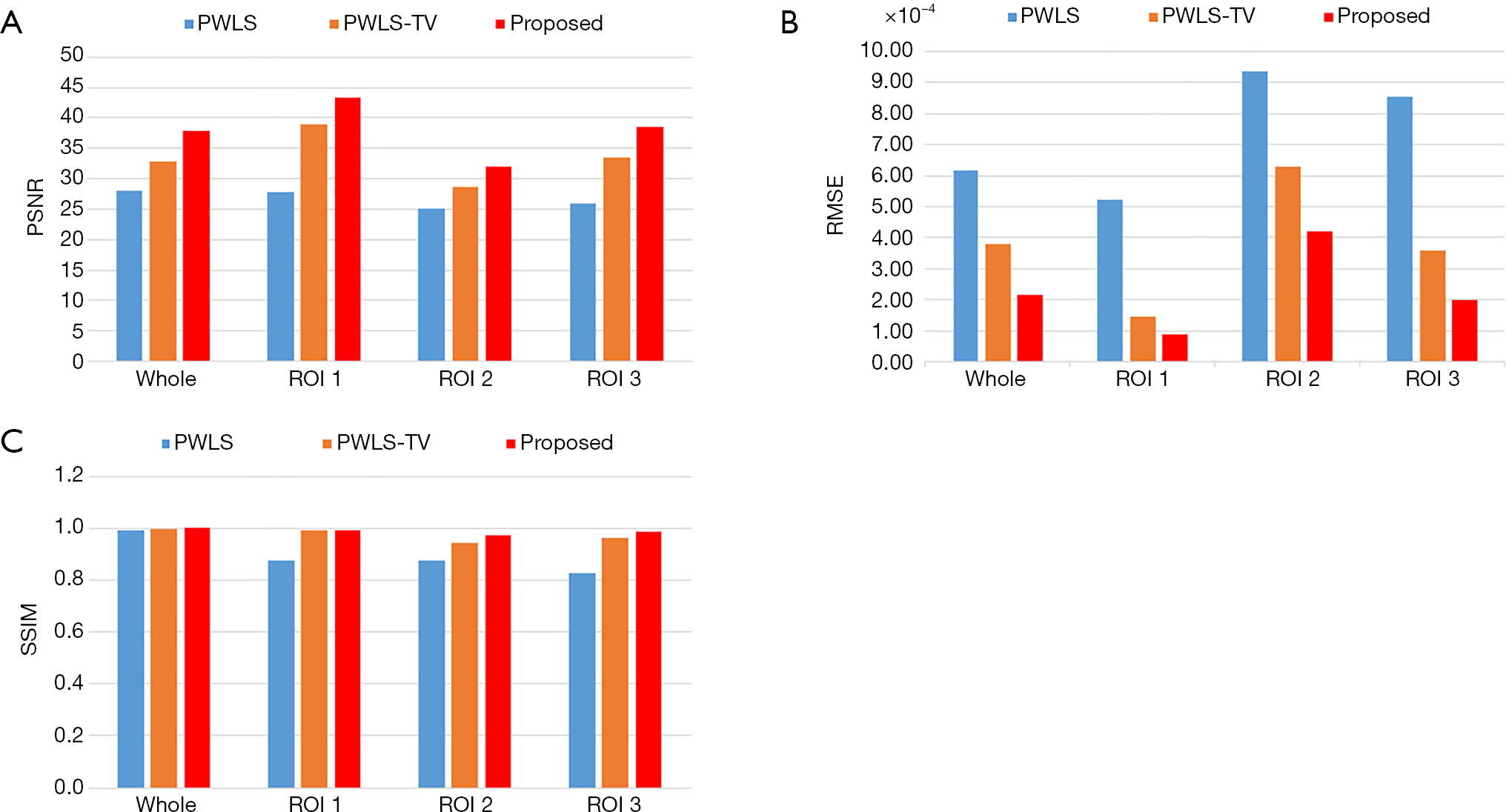Figure 7 Performance comparisons of the three algorithms on the whole image and on each ROI marked in Figure 5A. Bar charts of the PSNR, RMSE and SSIM values are shown in (A,B,C), respectively (for 90 sparse-view projections over 2π at an X-ray exposure level of I0 =5×106). PSNR, peak signal-to-noise ratio; RMSE, root mean square error; SSIM, structural similarity; ROI, regions of interest; PWLS, penalized weighted least squares; PWLS-TV, total variation based on penalized weighted least squares.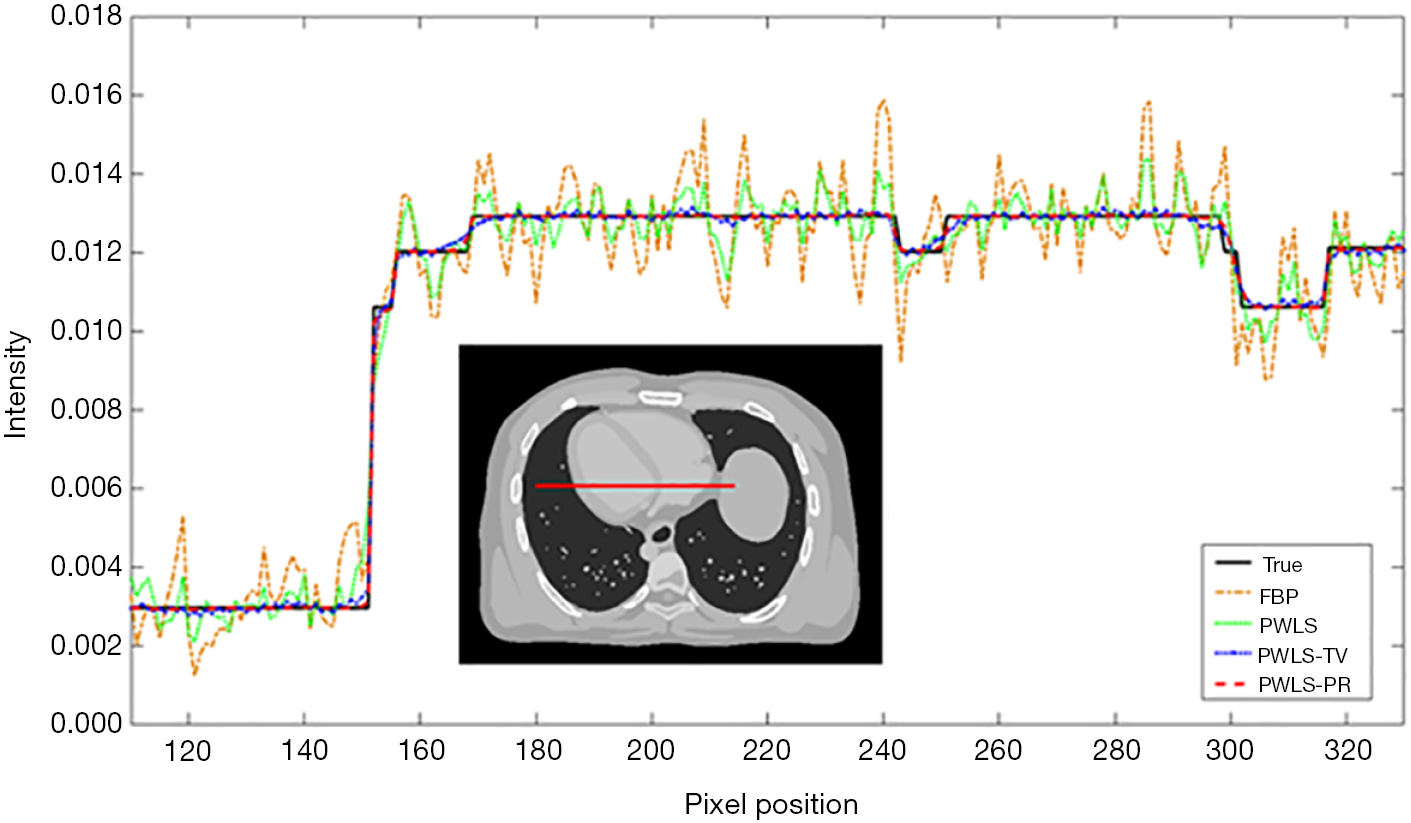Figure 8 Intensity profiles along the horizontal line (red) through the image (for 90 sparse-view projections over 2π at an X-ray exposure level of I0 =5×106). FBP, filtered back projection; PWLS, penalized weighted least squares; PWLS-TV, total variation based on penalized weighted least squares; PWLS-PR, patch-based regularization method based on penalized weighted least squares total variation.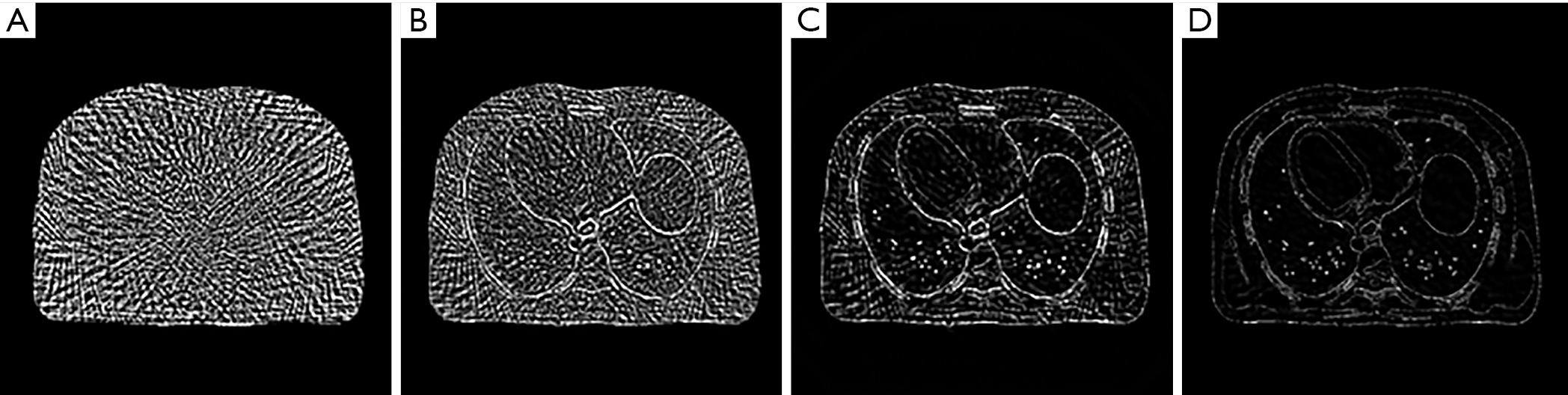Figure 9 Panels (A,B,C,D) show the residual images for the FBP, PWLS, PWLS-TV and PWLS-PR methods, respectively (for 90 sparse-view projections over 2π at an X-ray exposure level of I0 =5×106). FBP, filtered back projection; PWLS, penalized weighted least squares; PWLS-TV, total variation based on penalized weighted least squares; PWLS-PR, patch-based regularization method based on penalized weighted least squares total variation.

All the algorithms were implemented in MATLAB 2016 (MathWorks). The programs were run on a typical desktop computer equipped with an Intel i7-9700 CPU @ 3.00 GHz and 64.0 GB of RAM.

### Physical experiment

In this section, a diagnostic head phantom (Atom Max 711 HN, CIRS Inc., VA, USA) was used for a physical experiment. CT scanning was performed on our laboratory’s CT platform (Varex G-242, Varex Imaging Corporation, UT, USA) with an X-ray tube voltage of 120 kV and a current of 11 mA. During the experiments, a bowtie filter and an additional 0.5 mm copper filter were used. An energy-resolving photon counting detector (XC-Hydra FX50, XCounter AB, Sweden) with an imaging area of 512 mm × 6 mm and a native component size of 0.1 mm × 0.1 mm was used. The projection data were rebinned from 5,200×60 pixels to 850×10 pixels through the following steps:

• Discard the 10 columns of data collected at both the left and right edges of the detector panel;
• Rebin the dataset in a 6×6 binning mode.

The middle two layers of the rebinned 3D projection data were extracted and averaged to obtain the 2D data. The distance from the source to the center of gantry rotation was 1,000 mm, and the distance from the X-ray source to the detector was 1,500 mm. The gantry was rotated 360° with a 1° angular projection interval, and sparse-view data were obtained by under-sampling the whole set of 360 projection views to 60, 90, 120 and 180 views evenly over the original 360 views.

Similar to the simulation experiments, the image reconstructed from the projection data using the FBP approach with a ramp filter was used as the initial image. Figure 10 shows the images reconstructed from the ultra-low-dose sinogram data acquired at different sparse-view levels. From top to bottom, the numbers of views are 60, 90, 120, and 180. Figure 11 shows the images reconstructed from 90 sparse views using the different methods and the corresponding three zoomed-in ROIs. It is evident that our algorithm shows stronger robustness than the PWLS and PWLS-TV algorithms.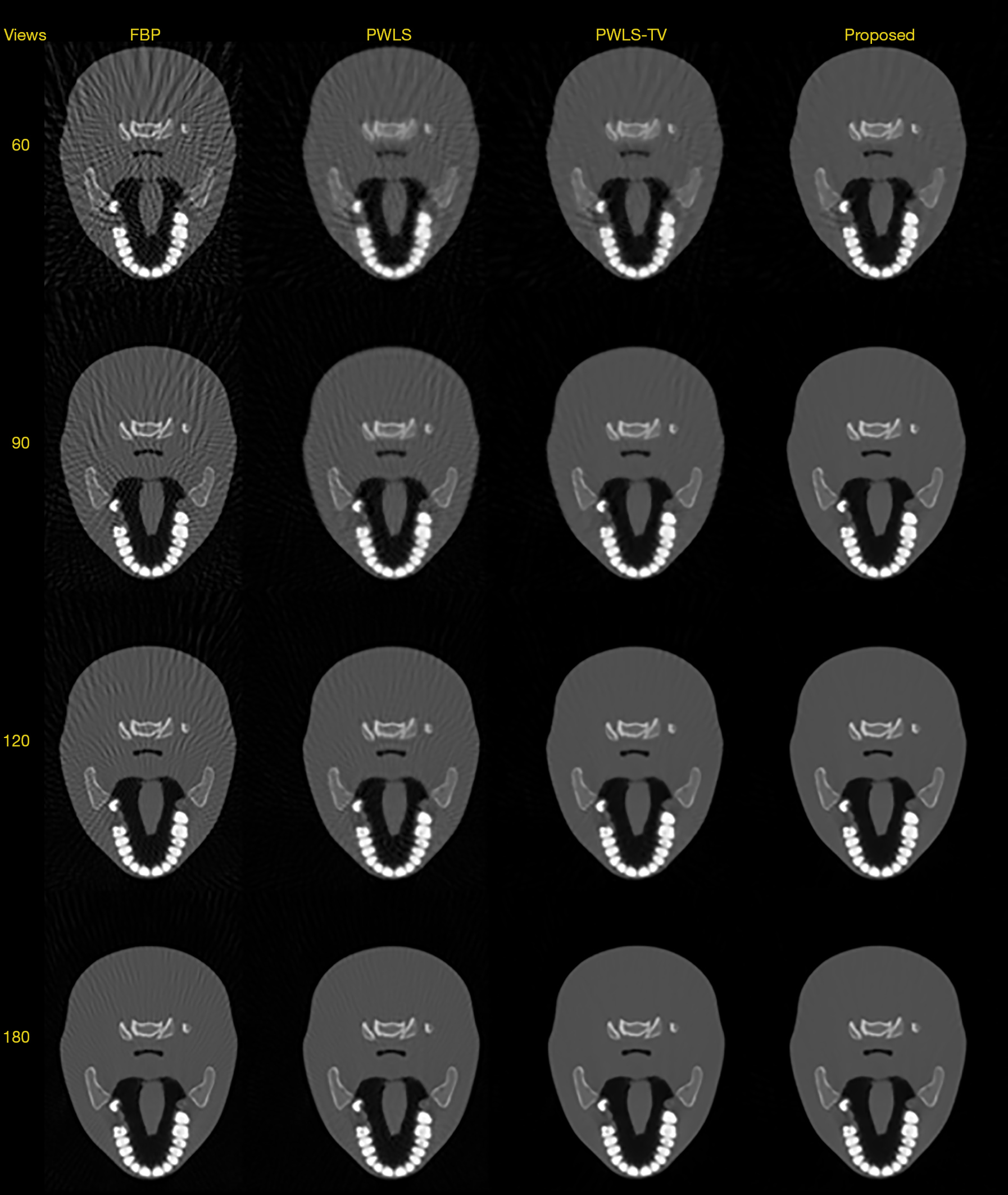Figure 10 Comparison of the performance of the three algorithms for the reconstruction of ROIs with detailed structures. These physical experimental results further prove that the PWLS-PR method achieves remarkable gains compared to the PWLS and PWLS-TV approaches in terms of preserving the structural information of the ROIs. FBP, filtered back projection; PWLS, penalized weighted least squares; PWLS-TV, total variation based on penalized weighted least squares; ROIs, regions of interest; PWLS-PR, patch-based regularization method based on penalized weighted least squares total variation.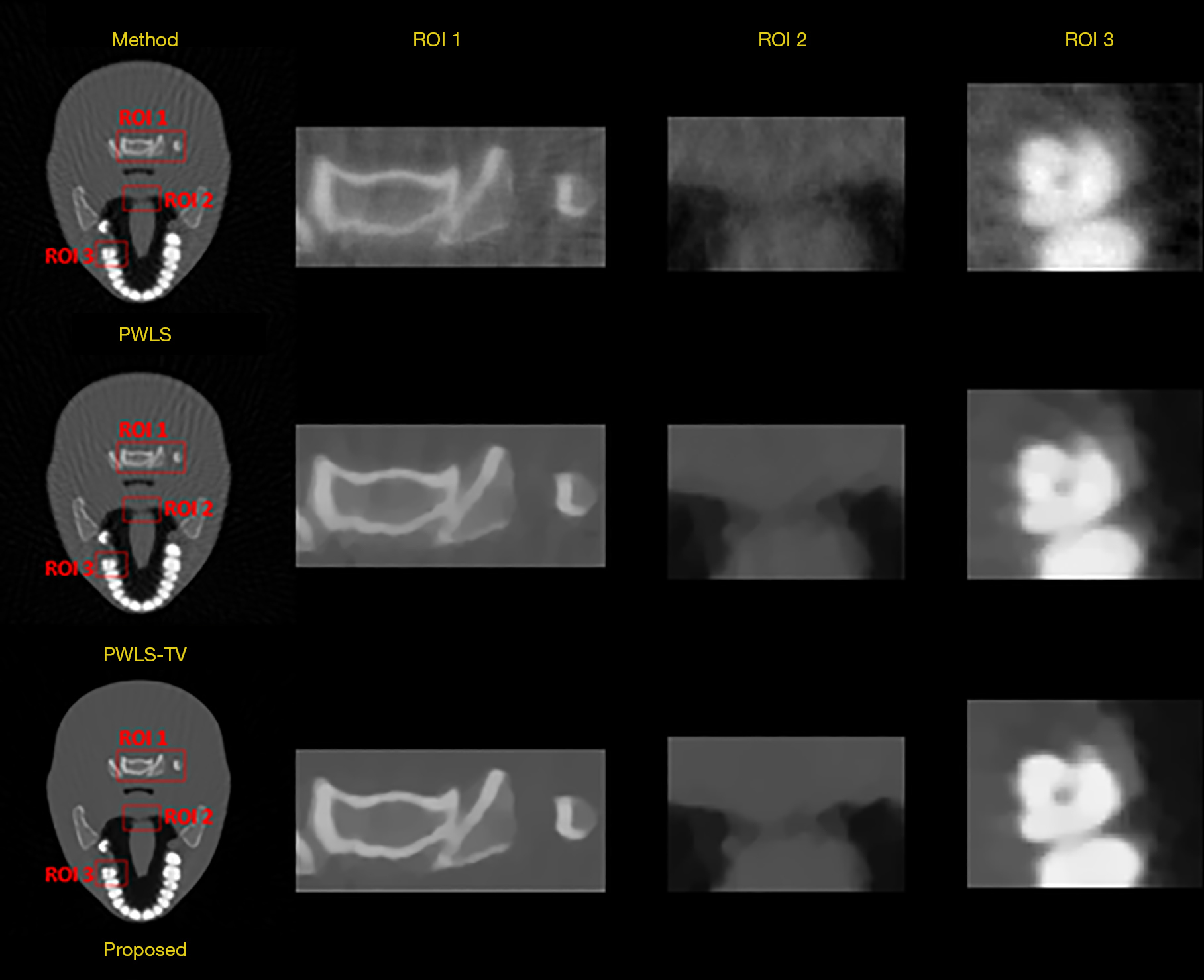Figure 11 Zoomed-in views of the three selected ROIs for the comparison of the PWLS, PWLS-TV and PWLS-PR approaches (for image reconstruction from 90 sparse-view projection data over 2π). FBP, filtered back projection; PWLS, penalized weighted least squares; PWLS-TV, total variation based on penalized weighted least squares; ROIs, regions of interest; PWLS-PR, patch-based regularization method based on penalized weighted least squares total variation.

## Discussion

In this work, we have proposed the novel PWLS-PR method with FBP sinogram restoration as a preprocessing step for ultra-low-dose image reconstruction from sinogram data acquired using a combined low X-ray current and sparse-view protocol. The PWLS-PR method was applied to reconstruct images from restored sinogram data.

In the implementation process, the weights of the PWLS-TV term were estimated using the nonlinear relationship between the mean and the variance and the mean values of the sinogram data. Noise reduction was achieved by manually selecting the hyperparameter β for PWLS-TV-based image reconstruction. In general, it is difficult to find a simple method to determine an appropriate value for β, and this value is usually chosen based on practical experience. In all of our experiments, we empirically found that a β value near 0.02 produced the best reconstructed images. Preliminary experimental results also showed that using the PWLS-TV method as the first step of the proposed method is useful for the reconstruction of ultra-low-dose CT images from data obtained using a combined low X-ray current and sparse-view protocol. However, it is worth noting that in such a protocol, when the X-ray current level and number of views are only moderately low, the results obtained via the PWLS-TV method seem to show an over-smoothing effect, which will reduce the resolution of the reconstructed image. Thus, how to reduce noise while maintaining the image resolution is a very important topic for discussion in ultra-low-dose image reconstruction.

Another parameter that requires attention is the hyperparameter δ in equation (15). The experimental results showed that the optimal range of δ is approximately 1×10−12 − 1×10−3. When δ is in the range of 1×10−12 − 1×10−3, it has little effect on the quality of the reconstructed images. Thus, in all of our experiments, we chose a δ value of 1×10−9.

The third parameter of interest is the patch size in equation (12). A larger patch size can lead to a slower runtime, and an excessively large patch size may hinder the recognition of small image features, resulting in the inability to preserve the corresponding edges. Based on our experience, we selected different patch sizes from 1×1 to 7×7 pixels, and we calculated the relative RMSE based on different patch size choices. The RMSE values for the different patch sizes are shown in Figure 12. Considering these results, we set the patch size to 3×3 pixels in our experiments.

The fourth parameter that may affect the results is the regularization parameter α in equation (9). A larger α value produces a smoother reconstructed image and reduces noise, but it also results in a lower resolution. How to determine the optimal α value for our proposed algorithm is an important question. Based on comprehensive consideration of the available options, in our experiments, we set α=0.01. Finally, the values and meanings of the parameters in the algorithm are summarized in Table 4.

## Conclusions

In this paper, we present a PWLS-PR method for sparse-view CT image reconstruction. Each iteration of the algorithm proposed in this paper can be described in the following three steps: image updating via the PWLS-TV, image smoothing, and pixel-by-pixel image fusion. This method has been validated by computer simulations and a physical experiment.

The experimental results demonstrate that the proposed PWLS-PR approach can preserve the detailed structure of the desired image. Compared with the existing PWLS and PWLS-TV methods, the PWLS-PR approach can achieve significant gains. However, as shown in Table 5, the PWLS-PR algorithm will increase the computational complexity due to its high efficiency. This issue is worth discussing in the future. Furthermore, this study shows that the PWLS-PR method reduces the amount of projection data required for repeated CT scans and has the useful potential to reduce the radiation dose in clinical medical applications.

## Acknowledgments

The authors would like to thank the editor and anonymous reviewers for their constructive comments and suggestions.

Funding: This work was supported by the Guangdong Special Support Program of China (2017TQ04R395), the Shenzhen International Cooperation Research Project of China (GJHZ20180928115824168), the Guangdong International Science and Technology Cooperation Project of China (2018A050506064), and the Natural Science Foundation of Guangdong Province in China (2020A1515010733).

## Footnote

Conflicts of Interest: All authors have completed the ICMJE uniform disclosure form (available at http://dx.doi.org/10.21037/qims-20-963). The authors have no conflicts of interest to declare.

Open Access Statement: This is an Open Access article distributed in accordance with the Creative Commons Attribution-NonCommercial-NoDerivs 4.0 International License (CC BY-NC-ND 4.0), which permits the non-commercial replication and distribution of the article with the strict proviso that no changes or edits are made and the original work is properly cited (including links to both the formal publication through the relevant DOI and the license). See: https://creativecommons.org/licenses/by-nc-nd/4.0/.

1. Zhang B, Zhang J, Chen H, Yang K, Zhang S. Unmatched clinical presentation and chest CT manifestation in a patient with severe coronavirus disease 2019 (COVID-19). Quant Imaging Med Surg 2020;10:871-3. [Crossref] [PubMed]
2. Jassel IS, Siddique M, Frost ML, Moore AEB, Puri T, Blake GM. The influence of CT and dual-energy X-ray absorptiometry (DXA) bone density on quantitative [(18)F] sodium fluoride PET. Quant Imaging Med Surg 2019;9:201-9. [Crossref] [PubMed]
3. Brenner DJ, Hall EJ. Computed tomography--an increasing source of radiation exposure. N Engl J Med 2007;357:2277-84. [Crossref] [PubMed]
4. Hsieh J. Adaptive streak artifact reduction in computed tomography resulting from excessive x-ray photon noise. Med Phys 1998;25:2139-47. [Crossref] [PubMed]
5. Kachelrieß M, Watzke O, Kalender WA. Generalized multi-dimensional adaptive filtering for conventional and spiral single-slice, multi-slice, and cone-beam CT. Med Phys 2001;28:475-90. [Crossref] [PubMed]
6. Moore CJ, Marchant TE, Amer AM. Cone beam CT with zonal filters for simultaneous dose reduction, improved target contrast and automated set-up in radiotherapy. Phys Med Biol 2006;51:2191-204. [Crossref] [PubMed]
7. Kalra MK, Wittram C, Maher MM, Sharma A, Avinash GB, Karau K, Toth TL, Halpern E, Saini S, Shepard JA. Can Noise Reduction Filters Improve Low-Radiation-Dose Chest CT Images? Pilot Study. Radiology 2003;228:257-64. [Crossref] [PubMed]
8. McCollough CH, Primak AN, Braun N, Kofler J, Yu L, Christner J. Strategies for reducing radiation dose in CT. Radiol Clin North Am 2009;47:27-40. [Crossref] [PubMed]
9. Maruhashi T, Morita H, Arimoto M, Kataoka J, Fujieda K, Nitta H, Ikeda H, Kiji H. Evaluation of a novel photon-counting CT system using a 16-channel MPPC array for multicolor 3-D imaging. Nucl Instrum Methods Phys Res A 2019;936:5-9. [Crossref]
10. Kalender WA, Wolf H, Suess C, Gies M, Greess H, Bautz WA. Dose reduction in CT by on-line tube current control: principles and validation on phantoms and cadavers. Eur Radiol 1999;9:323-8. [Crossref] [PubMed]
11. McCollough CH, Bruesewitz MR, Kofler JM. CT Dose Reduction and Dose Management Tools: Overview of Available Options. RadioGraphics 2006;26:503-12. [Crossref] [PubMed]
12. Hu Z, Jiang C, Sun F, Zhang Q, Ge Y, Yang Y, Liu X, Zheng H, Liang D. Artifact correction in low-dose dental CT imaging using Wasserstein generative adversarial networks. Med Phys 2019;46:1686-96. [Crossref] [PubMed]
13. Gu P, Jiang C, Ji M, Zhang Q, Ge Y, Liang D, Liu X, Yang Y, Zheng H, Hu Z. Low-Dose Computed Tomography Image Super-Resolution Reconstruction via Random Forests. Sensors (Basel) 2019;19:207. [Crossref] [PubMed]
14. Smith AB, Dillon WP, Gould R, Wintermark M. Radiation dose-reduction strategies for neuroradiology CT protocols. AJNR Am J Neuroradiol 2007;28:1628-32. [Crossref] [PubMed]
15. Wang S, Wu W, Feng J, Liu F, Yu H. Low-dose spectral CT reconstruction based on image-gradient L0-norm and adaptive spectral PICCS. Phys Med Biol 2020;65:245005 [Crossref] [PubMed]
16. Xu M, Hu D, Luo F, Liu F, Wang S, Wu W. Limited-Angle X-Ray CT Reconstruction Using Image Gradient ℓ0-Norm With Dictionary Learning. IEEE Trans Radiat Plasma Med Sci 2021;5:78-87. [Crossref]
17. Wu W, Hu D, An K, Wang S, Luo F. A High-Quality Photon-Counting CT Technique Based on Weight Adaptive Total-Variation and Image-Spectral Tensor Factorization for Small Animals Imaging. IEEE Trans Instrum Meas 2020;70:2502114
18. Kawashima H, Ichikawa K, Takata T, Mitsui W. Algorithm-based artifact reduction in patients with arms-down positioning in computed tomography. Physica Medica 2020;69:61-9. [Crossref] [PubMed]
19. Wu W, Chen P, Wang S, Vardhanabhuti V, Liu F, Yu H. Image-domain Material Decomposition for Spectral CT using a Generalized Dictionary Learning. IEEE Trans Radiat Plasma Med Sci 2020; [Crossref]
20. Bouman CA, Sauer K. A unified approach to statistical tomography using coordinate descent optimization. IEEE Trans Image Process 1996;5:480-92. [Crossref] [PubMed]
21. Elbakri IA, Fessler JA. Statistical image reconstruction for polyenergetic X-ray computed tomography. IEEE Trans Med Imaging 2002;21:89-99. [Crossref] [PubMed]
22. Thibault JB, Sauer KD, Bouman CA, Hsieh J. A three-dimensional statistical approach to improved image quality for multislice helical CT. Med Phys 2007;34:4526-44. [Crossref] [PubMed]
23. Ouyang L, Solberg T, Wang J. Effects of the penalty on the penalized weighted least-squares image reconstruction for low-dose CBCT. Phys Med Biol 2011;56:5535-52. [Crossref] [PubMed]
24. Xu Q, Yu H, Mou X, Zhang L, Hsieh J, Wang G. Low-Dose X-ray CT Reconstruction via Dictionary Learning. IEEE Trans Med Imaging 2012;31:1682-97. [Crossref] [PubMed]
25. Tang S, Tang X. Statistical CT noise reduction with multiscale decomposition and penalized weighted least squares in the projection domain. Med Phys 2012;39:5498-512. [Crossref] [PubMed]
26. Lauzier PT, Chen G-H. Characterization of statistical prior image constrained compressed sensing (PICCS): II. Application to dose reduction. Med Phys 2013;40:021902 [Crossref] [PubMed]
27. Hu Z, Zhang Y, Liu J, Ma J, Zheng H, Liang D. A feature refinement approach for statistical interior CT reconstruction. Phys Med Biol 2016;61:5311-34. [Crossref] [PubMed]
28. Hu Z, Gui J, Zou J, Rong J, Zhang Q, Zheng H, Xia D. Geometric Calibration of a Micro-CT System and Performance for Insect Imaging. IEEE Trans Inf Technol Biomed 2011;15:655-60. [Crossref] [PubMed]
29. Zhang S, Zhou P, Pan K, Liu Z, Li Y. A SART algorithm for area integral model in desktop micro-CT system. Nucl Instrum Methods Phys Res A 2020;955:163288 [Crossref]
30. Wang J, Li T, Lu H, Liang Z. Penalized weighted least-squares approach to sinogram noise reduction and image reconstruction for low-dose X-ray computed tomography. IEEE Trans Med Imaging 2006;25:1272-83. [Crossref] [PubMed]
31. Ma J, Zhang H, Gao Y, Huang J, Liang Z, Feng Q, Chen W. Iterative image reconstruction for cerebral perfusion CT using a pre-contrast scan induced edge-preserving prior. Phys Med Biol 2012;57:7519-42. [Crossref] [PubMed]
32. Yu DF, Fessler JA. Edge-preserving tomographic reconstruction with nonlocal regularization. IEEE Trans Med Imaging 2002;21:159-73. [Crossref] [PubMed]
33. Sidky EY, Kao CM, Pan X. Accurate image reconstruction from few-views and limited-angle data in divergent-beam CT. J Xray Sci Technol 2006;14:119-39.
34. LaRoque SJ, Sidky EY, Pan X. Accurate image reconstruction from few-view and limited-angle data in diffraction tomography. J Opt Soc Am A Opt Image Sci Vis 2008;25:1772-82. [Crossref] [PubMed]
35. Huang J, Ma J, Liu N, Zhang H, Bian Z, Feng Y, Feng Q, Chen W. Sparse angular CT reconstruction using non-local means based iterative-correction POCS. Comput Biol Med 2011;41:195-205. [Crossref] [PubMed]
36. Sidky EY, Pan X. Image reconstruction in circular cone-beam computed tomography by constrained, total-variation minimization. Phys Med Biol 2008;53:4777-807. [Crossref] [PubMed]
37. Tian Z, Jia X, Yuan K, Pan T, Jiang SB. Low-dose CT reconstruction via edge-preserving total variation regularization. Phys Med Biol 2011;56:5949-67. [Crossref] [PubMed]
38. Liu Y, Ma J, Fan Y, Liang Z. Adaptive-weighted total variation minimization for sparse data toward low-dose x-ray computed tomography image reconstruction. Phys Med Biol 2012;57:7923-56. [Crossref] [PubMed]
39. Zhang Y, Huang J, Ma J, Zhang H, Bian Z, Zeng D, Gao Y, Chen W. Iterative image reconstruction for ultra-low-dose CT with a combined low-mAs and sparse-view protocol. Annu Int Conf IEEE Eng Med Biol Soc 2013;2013:5107-10. [PubMed]
40. Wang G, Qi J. Penalized Likelihood PET Image Reconstruction Using Patch-Based Edge-Preserving Regularization. IEEE Trans Med Imaging 2012;31:2194-204. [Crossref] [PubMed]
41. Tahaei MS, Reader AJ. Patch-based image reconstruction for PET using prior-image derived dictionaries. Phys Med Biol 2016;61:6833-55. [Crossref] [PubMed]
42. Gilboa G, Osher S. Nonlocal Linear Image Regularization and Supervised Segmentation. Multiscale Model Simul 2007;6:595-60. [Crossref]
43. Kervrann C, Boulanger J. Optimal Spatial Adaptation for Patch-Based Image Denoising. IEEE Trans Image Process 2006;15:2866-78. [Crossref] [PubMed]
44. Zhang W, Gao J, Yang Y, Liang D, Liu X, Zheng H, Hu Z. Image reconstruction for positron emission tomography based on patch-based regularization and dictionary learning. Med Phys 2019;46:5014-26. [Crossref] [PubMed]
45. Lu Y, Zhao J, Wang G. Few-view image reconstruction with dual dictionaries. Phys Med Biol 2012;57:173-89. [Crossref] [PubMed]
46. Chang H, Yeung DY, Xiong Y. Super-resolution through neighbor embedding. Proceedings of the 2004 IEEE Computer Society Conference on Computer Vision and Pattern Recognition, 2004. CVPR 2004. 27 June-2 July 2004; Washington, DC, USA. IEEE, 2004.
47. Yang J, Wright J, Huang T, Ma Y. Image super-resolution as sparse representation of raw image patches. 2008 IEEE Conference on Computer Vision and Pattern Recognition. 23-28 June 2008; Anchorage, AK, USA. IEEE, 2008.
48. Huang J, Zhang Y, Ma J, Zeng D, Bian Z, Niu S, Feng Q, Liang Z, Chen W. Iterative Image Reconstruction for Sparse-View CT Using Normal-Dose Image Induced Total Variation Prior. PLoS One 2013;8:e79709 [Crossref] [PubMed]
49. Lange K. Convergence of EM image reconstruction algorithms with Gibbs smoothing. IEEE Trans Med Imaging 1990;9:439-46. [Crossref] [PubMed]
50. Erdoğan H, Fessler JA. Monotonic algorithms for transmission tomography. IEEE Trans Med Imaging 1999;18:801-14. [Crossref] [PubMed]
51. Ma J, Feng Q, Feng Y, Huang J, Chen W. Generalized Gibbs priors based positron emission tomography reconstruction. Comput Biol Med 2010;40:565-71. [Crossref] [PubMed]
52. Segars WP, Sturgeon G, Mendonca S, Grimes J, Tsui BMW. 4D XCAT phantom for multimodality imaging research. Med Phys 2010;37:4902-15. [Crossref] [PubMed]
53. La Rivière PJ, Billmire DM. Reduction of noise-induced streak artifacts in X-ray computed tomography through spline-based penalized-likelihood sinogram smoothing. IEEE Trans Med Imaging 2005;24:105-11. [Crossref] [PubMed]
54. Wang Z, Bovik AC, Sheikh HR, Simoncelli EP. Image quality assessment: from error visibility to structural similarity. IEEE Trans Image Process 2004;13:600-12. [Crossref] [PubMed]
Cite this article as: Fu J, Feng F, Quan H, Wan Q, Chen Z, Liu X, Zheng H, Liang D, Cheng G, Hu Z. PWLS-PR: low-dose computed tomography image reconstruction using a patch-based regularization method based on the penalized weighted least squares total variation approach. Quant Imaging Med Surg 2021;11(6):2541-2559. doi: 10.21037/qims-20-963